Mobile QR Code1. (Dept. of Electronics and Communication Engineering, Hanyang University, Ansan 15588, Korea)

Programmable memory built-In self-test (PMBIST) margin test, DDR4 I/O timing margins, pseudo-random binary sequence (PRBS), inter-connect fault model, fault-critical-random-94 (FCR-94) data pattern (DP) set

## I. INTRODUCTION

As semiconductor process technology scales down, the integration density of DRAMs has rapidly increased. Due to the scaling, DRAM cells are more apt to induce errors as they get closer to each other. The eye of DRAM data lines (DQs) is reduced because of the reduced I/O voltage (VDDQ). The noise due to the reduction of inter-signal space and higher I/O speed, such as crosstalk, jitter, inter-symbol interference (ISI), and power delivery network (PDN) noise, can exacerbate the eye even more and cause a DRAM to malfunction. Even though many innovative techniques have been adopted to control the signal integrity issue for today’s DRAMs (1-3), noise effects get worse as the contemporary DRAM is operating at speeds up to 3200 Mbps. Therefore, DRAM I/O timing margins are the critical and effective metric to evaluate the combined effects of various noise issues.

Several studies for timing margins have been published. Timing margins of t$_{\mathrm{AC}}$ and t$_{\mathrm{DQSCK}}$ from DDR2 DRAM were measured in (4). By using the automatic test equipment (ATE), the conditions of yielding the worst-case timing margins were easily investigated. In (5), the authors showed that the timing margins in DDR3 RDIMMs could be improved by changing several on-die termination (ODT) settings; they changed the READ ODT value and it improved the timing margins for a single DQ. They also modified the ODT firing sequence and it improved hold margins of DQs. They also suggested several modifications of timing parameters to enhance the timing margins. In (6), the authors describe the use of voltage reference (VREF) training to find optimal timing margins in DDR4 RDIMMs. In DDR4, a new feature called per DRAM addressability (PDA) was introduced, making it possible to implement a trainable internal reference voltage (VREFDQ) to optimize the timing margins for each DRAM chip at acceptable power consumption levels. Time interval error (TIE) histograms of various generated data patterns were presented in (7). These data patterns included one or more noise sources based on interconnect fault models, and they were employed to understand the influence of noise on the timing margins.

In this paper, we report the sensitivity of three test pattern factors such as test algorithms, address directions (ADs), and data patterns (DPs) on I/O timing margins. In this work, programmable memory built-in self-test (PMBIST) is implemented to configure test pattern factors. We can observe direct consequences in timing margins for multiple test pattern factors that can be configured and controlled by a software interface.

In general, it is reported that the pseudo-random binary sequence (PRBS) DPs are quite useful in evaluating I/O characteristics, and they are used for the determination of eye diagrams of I/Os (8). PRBS DPs have been used in many areas such as I/O characteristic analysis and stress injection (8-11).

In this work, we experimentally demonstrated that there could be a small set of random patterns out of the entire PRBS DP set, and selected random patterns can produce equivalent margin to the entire PRBS DP set when they are repeated. In addition to the pruning PRBS patterns, the fault-based deterministic patterns are also developed. By experimentally selecting critical random patterns and intelligently taking advantage of both random and deterministic DPs, we confirmed that timing margins could be aggressively stressed out, which was not achievable using either blindly employing random or deterministic patterns alone.

This paper is organized as follows. Section 2 explains the system configuration and the margin test methodology used for the margin test. In Section 3, test pattern factors used in the margin tests are described. Section 4 introduces the effects on timing margins after changing each test pattern factor and keeping other factors the same. In Section 5, it is experimentally demonstrated that some critical random and fault-based deterministic DPs make a worse impact in terms of timing margins than the PRBS DP set. Section 6 ends with a conclusion.

Fig. 1. A block diagram of the memory tester for measuring timing margins.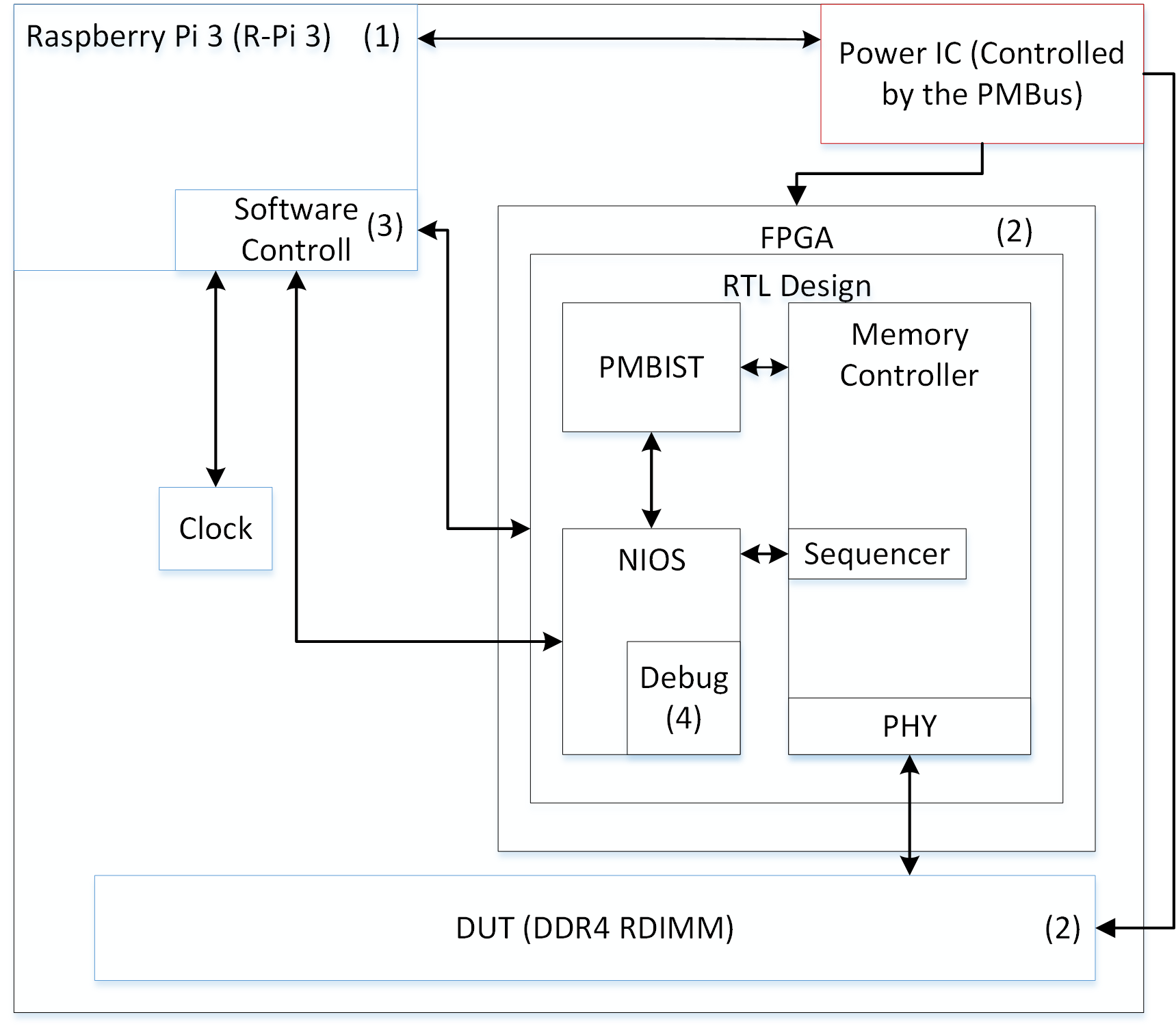## II. PMBIST Margin Test

### 1. System Configuration

Fig. 1 shows a block diagram of the memory test environment to perform PMBIST margin tests for DDR4 RDIMM sample. For experiments, all Rank 0 addresses (28 bits) of the DDR4 RDIMM were sequentially and randomly sequenced at the high speed of 2133 Mbps during timing margin measurements.

The various test patterns were generated by using the PMBIST engine and its associated software. The PMBIST based margin test has the advantage of measuring eyes of all data lines (DQs) and data strobes (DQSs).

The memory tester is rack-mountable, making it convenient to stack as many memory testers as needed to perform multiple tests in parallel.

The hardware initialization sequence for performing margin tests is as follows (refer to Fig. 1). (1) A user applies power through a Power IC to bring up a Raspberry Pi (R-Pi). Users can access the R-Pi remotely and can manipulate test pattern factors such as selecting test algorithms and DPs. (2) The Power IC supplies power to the FPGA and RDIMM. (3) The R-Pi configures the clock frequency. After that, the R-Pi loads a register-transfer level (RTL) design into FPGA, and the RTL design includes a memory controller, a PMBIST, and a Nios. The RDIMM calibration sequence is executed during the loading process. (4) After initialization in steps 1 ${-}$ 3, the hardware is ready for margin tests.

Fig. 2. The methods of measuring (a) setup margin, (b) hold margin.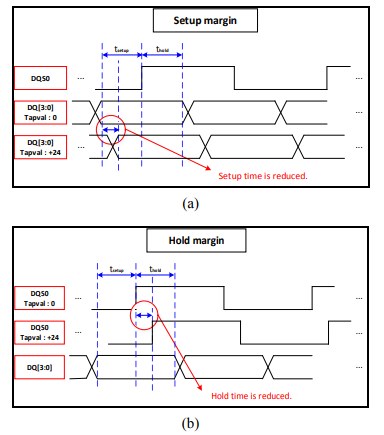### 2. Margin Test Methodology

Fig. 2 shows timing diagrams of DQS and DQ to illustrate setup and hold margins. In Fig. 2, the DQS rising edge is aligned at the center of DQs after the calibration. In this state, the DQs are located at the zero-tap value. The “tap value” is used to describe the relative location of the DQ and DQS. When the DQs are delayed from the original time, e.g., the DQs are delayed by the tap value of 24 (refer to Fig. 2(a)), the setup time will be reduced. We can measure the setup margin by continuously delaying the DQs until a failure is detected. Conversely, the hold margin can be measured by continuously delaying the DQS until a failure is detected (refer to Fig. 2(b)).

There are two types of taps, read and write taps, for the purposes of measuring two kinds of margins. The read tap is associated with a read delay path in the memory controller, so that the DQS and DQ signals from the memory will be delayed when a read operation occurs. In the case of the write tap, which is linked to a write delay path, the delayed DQS and DQ signals will be sent to the memory when a write operation occurs. The tap value indicates how much the signals will be delayed.

Two kinds of timing margins are examined in this paper: a read margin and a write margin. The read margin is defined as the sum of the setup and hold margins measured by using read taps. Likewise, the write margin is defined as the total amount of the setup and hold margins measured by using write taps.

## III. Test Pattern Factors

### 1. Test Algorithms and Address Directions

Table 1 shows the test algorithms used during the margin tests. The detail explanations of march operations, address orders, and march elements, are described in (12).

The time complexities of MSCAN and March C- are 4n and 10n, respectively. In moving inversion (MOVI), the address increases by 2$^{\mathrm{r}}$ with a carry, where r = 0, 1, 2, $\ldots$, total tested address bits - 1. Since we tested whole addresses of Rank 0, a total of 28 address bits were used in the margin test. After the test was finished with r = 0 (address increment by 1), the test was started again with r = 1 (address increment by 2), and the test continued until r reached 28. The time complexity of the MOVI algorithm is thus 168n, which is considerably long. Note that the MOVI introduced in (12) was longer than we used in this paper, but we simplified it to stress address rotations and to reduce the test time.

The test algorithms were executed with two ADs in the margin tests. The associated ADs were (1) Numerical and (2) PRBS. In the Numerical AD, the addresses were numerically increasing or decreasing. In the PRBS AD, address increment/decrement followed a pseudo-random binary sequence created by a linear feedback shift register (13). Due to unknown scrambling information (14), we were not able to factor in the physical structure in margin test.

### 2. Data Patterns

One DP and two DP sets were used by the test algorithms. A DP set consists of a number of DPs. The DP of all-DQ toggling, and the DP sets of PRBS and fault-based were used. In the all-DQ toggling DP, all-zeros (0000$\ldots$) were written at the even burst write cycles and all-ones (1111$\ldots$) were written at odd burst write cycles. Since all 72 DQs are simultaneously switching at each data cycle, large power consumption is expected to occur to affect the timing margins through the power noise.

The fault-based DP set was generated to cover all interconnect fault models considered in (7). When a target DQ was selected to generate the noise-included patterns, the neighborhoods of the target DQ were considered based on the physically adjacent DQs from the physical perspectives of the RDIMM or the FPGA. The patterns were generated until all 72 DQs were considered as targets. The generated DP in the set covered one or more fault models. Thus, it was expected to observe how each or combined noise sources affected the timing margin.

The PRBS DP set was adopted to invoke random noise effects on the timing margins. Many noise sources could be blended in the PRBS DP set, such as ISI, SSN, crosstalk, and PDN noise, that would be mimicking the situation when the functional operation was conducted. Such random effect is not practically possible with the fault model-based approaches.

## IV. Timing Margins according to Test Pattern Factors

In this section, we compare timing margins for different test pattern factors. The timing margins are measured for each DQS group (DG). Since the setup margin is acquired by delaying each DQ signal, the setup margin of a corresponding DG is defined as the minimum of the DQ setup margins that are affected by the same DQS. On the other hand, hold margins are measured by delaying DQSs. Thus, the read or write margin of a DG is defined by adding the hold margin to the setup margin defined above.

Fig. 3. Comparison for (a) read margins, (b) write margins depending on the test algorithms.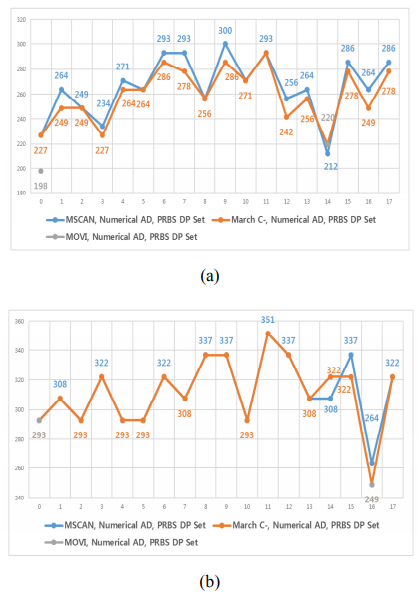### 1. Changes due to Test Algorithms

Fig. 3 shows (a) the read margins and (b) the write margins for three test algorithms. The test algorithms used for comparison were the MSCAN, March C-, and MOVI (12). The Numerical AD and the PRBS DP set were applied for these test algorithms. For the rest of the figures, the X-axis stands for DGs, and Y-axis shows timing margins (unit: ps) for the corresponding DGs. The timing margin has the resolution of 7.3 ps for the read margin and 14.6 ps for the write margin.

From Fig. 3, it is observed that timing margins were not the same for all DGs. This might be the result of process variations and the I/O timing adjustment made by DG during manufacturing. When comparing read and write margins on the same test algorithm, the DG (DG 9) with the maximum read margin is not same with the DG (DG 11) with the maximum write margin. The same is true of DGs for the minimum timing margin.

The maximum difference of the margin in MSCAN was 87.9 ps for both the read margin and the write margin. In the case of the read margin, the average margin of March C- was 2.3% (6.1 ps) less than that of MSCAN; it was observed that the margin was reduced by up to 14.6 ps (DG 1, 7, 12, 16). For the write margin, there was little difference between them. Decreases of 14.6 ps were observed at DG 15 and 16, whereas an increase of 14.6 ps was observed at DG 14.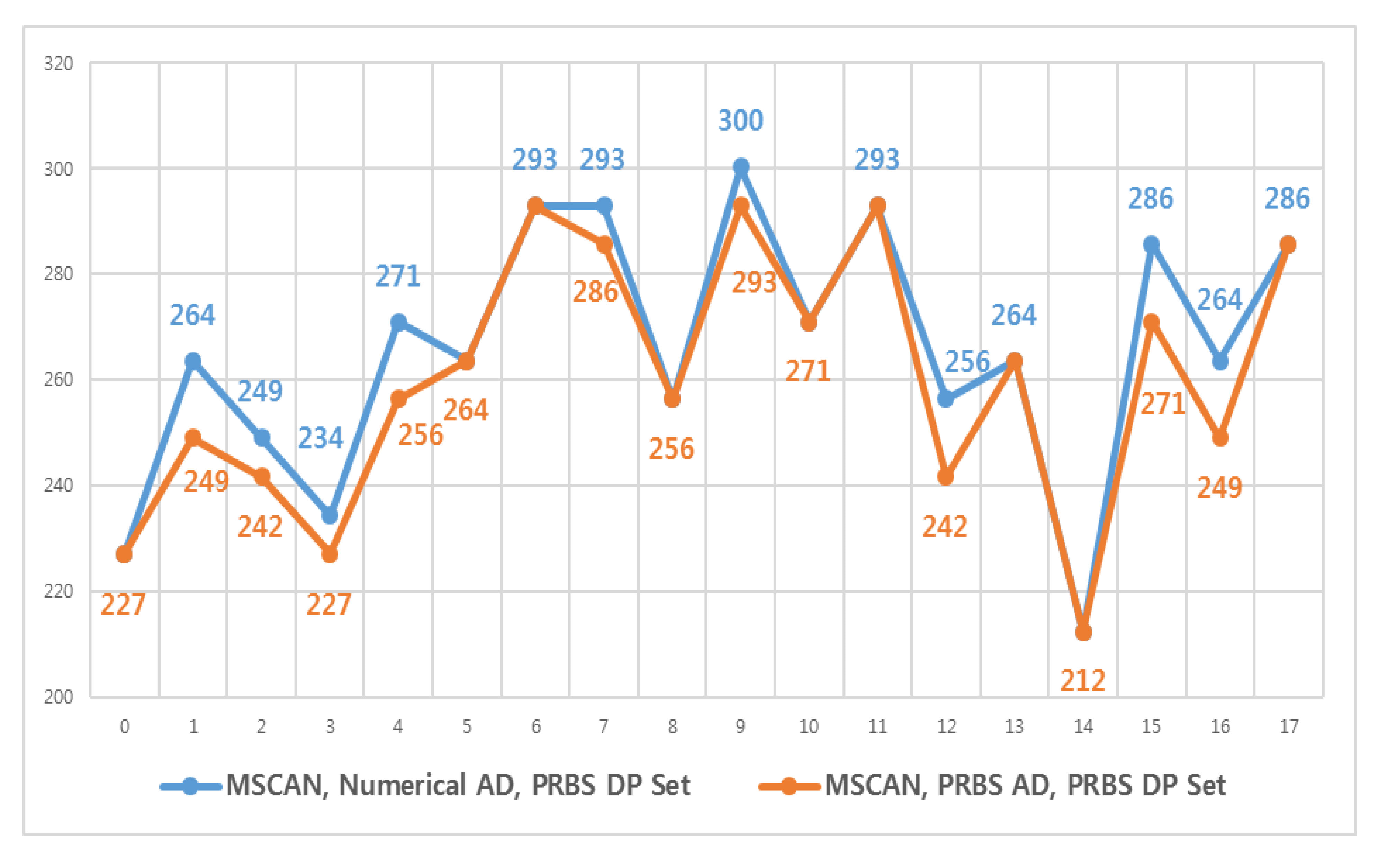In MOVI, only read margins for DG 0 and 14, and write margins for DG 0 and 16 were measured due to the long test time. Only DG 0 of the read margin was reduced by 12.8\% (29.3 ps), however, the margins of other measured DGs were very similar to those measured in other test algorithms. From these observations, the MOVI based margins for remaining DGs are expected to be similar to March C- (or MSCAN).

The time complexity of the test algorithm increased by 150% (4100%) when changed from MSCAN to March C- (MOVI). However, the maximum reduction of read margin was only 14.6 ps (29.3 ps for MOVI). Hence, we can conclude that test algorithms alone have little impact on timing margins.

### 2. Changes due to Address Directions

Fig. 4 compares the read margin results for MSCAN algorithm with two different ADs to see the sensitivity of AD on timing margins. In the PRBS AD, there are frequent row address changes and it increases the frequency of Activate-Precharge commands (2). Thus, it is expected to consume more power, so the timing margins would be lower than those of the Numerical AD.

Indeed, the PRBS AD used 8.2% more power during read margin (8% for write margin) measurements, however, the average reduction with the PRBS AD was only 2.1% (5.7 ps) for the read margin. The maximum reduction of 14.6 ps was observed (DG 1, 12, 15, 16). Write margins are not shown because there was no difference observed between two ADs. Thus, we can conclude that the effect of employing different ADs was insignificant to the timing margins.

Fig. 5. Comparison for (a) read margins, (b) write margins depending on the data patterns.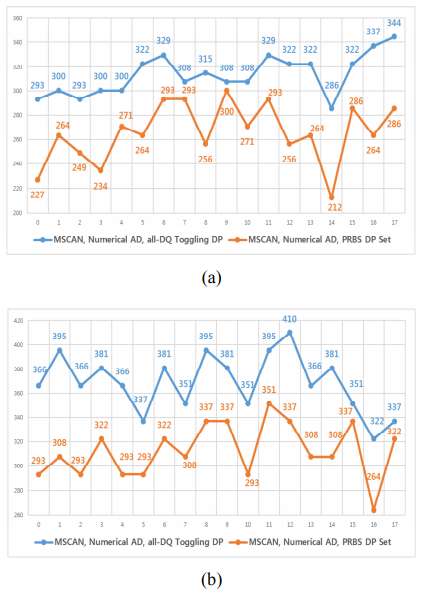### 3. Changes due to Data Patterns

Fig. 5 shows the effect of DPs on timing margins. The experiments were performed with the same MSCAN algorithm and Numerical AD by choosing either the all-DQ toggling DP or the PRBS DP set.

Among the DGs, the gap between minimum and maximum margin in the all-DQ toggling DP was 58.6 ps for the read margin and 87.9 ps for the write margin. The DGs, which showed the minimum timing margins, were the same for both the read (DG 14) and the write margin (DG 16) for two different DPs. Most sensitive DGs for both read and write operations were preserved irrespective of DPs.

In the case of the read margin, the average reduction of the PRBS DP set with respect to the all-DQ toggling DP was 15.2% (47.6 ps). The maximum decrement of 65.9 ps was observed (DG 0, 3, 12). For the write margin, it was decreased by 15.2% (56.1 ps) on average. The maximum reduction of 87.9 ps was measured at DG 1. Regardless of the types of timing margins, the margins were reduced at all DGs as the PRBS DP set was employed.

The all-DQ toggling DP consumed 0.9% more power during read margin (1.7% for write margin) measurements compared to the PRBS DP set. We could convince that a slight increase in power consumption insignificantly affected the timing margin (refer to Section 4.2). These results demonstrate that I/O timing margins are most sensitive to DPs.

## V. Further Analysis of The Effect of Data Patterns on Timing Margins

Throughout the experiments, we found that timing margins were strongly dependent on the DPs. Since all the PRBS DPs did not equally affect the timing margins, we performed margin tests to better understand the influence of a small number of powerful DPs on the margins. We collected 32 failed-DPs from each DQ or DQS test results and generated 62 DPs which were known as noise-included DPs (7), thus, the total number of DPs was 94. We ran margin tests with these DPs using reduced address bits to save the test time.

### 1. Random Pattern Candidate Selection

The experimental results described in Section 4 indicate that timing margins are strongly dependent on DPs. We conducted an experiment to discover which PRBS DPs had the largest effect on timing margins. We performed margin tests on some DQs using the first fail-detected DP from the PRBS DP set. The results showed two types of outcomes: DQs that showed same and DQs that showed similar results as those of the PRBS DP set.

From these experiments, we concluded that testing with a single DP for a large number of addresses might result in a lower timing margin than performing tests with a large number of DPs for all different addresses. We expected the timing margins could deteriorate further if more first-failed DPs were collected and tested. Thus, we collected the first 32 failed-DPs from the test results of each DQ or DQS.

Fig. 6. Comparison for read margins between the PRBS DP set and the FCR-94 DP set.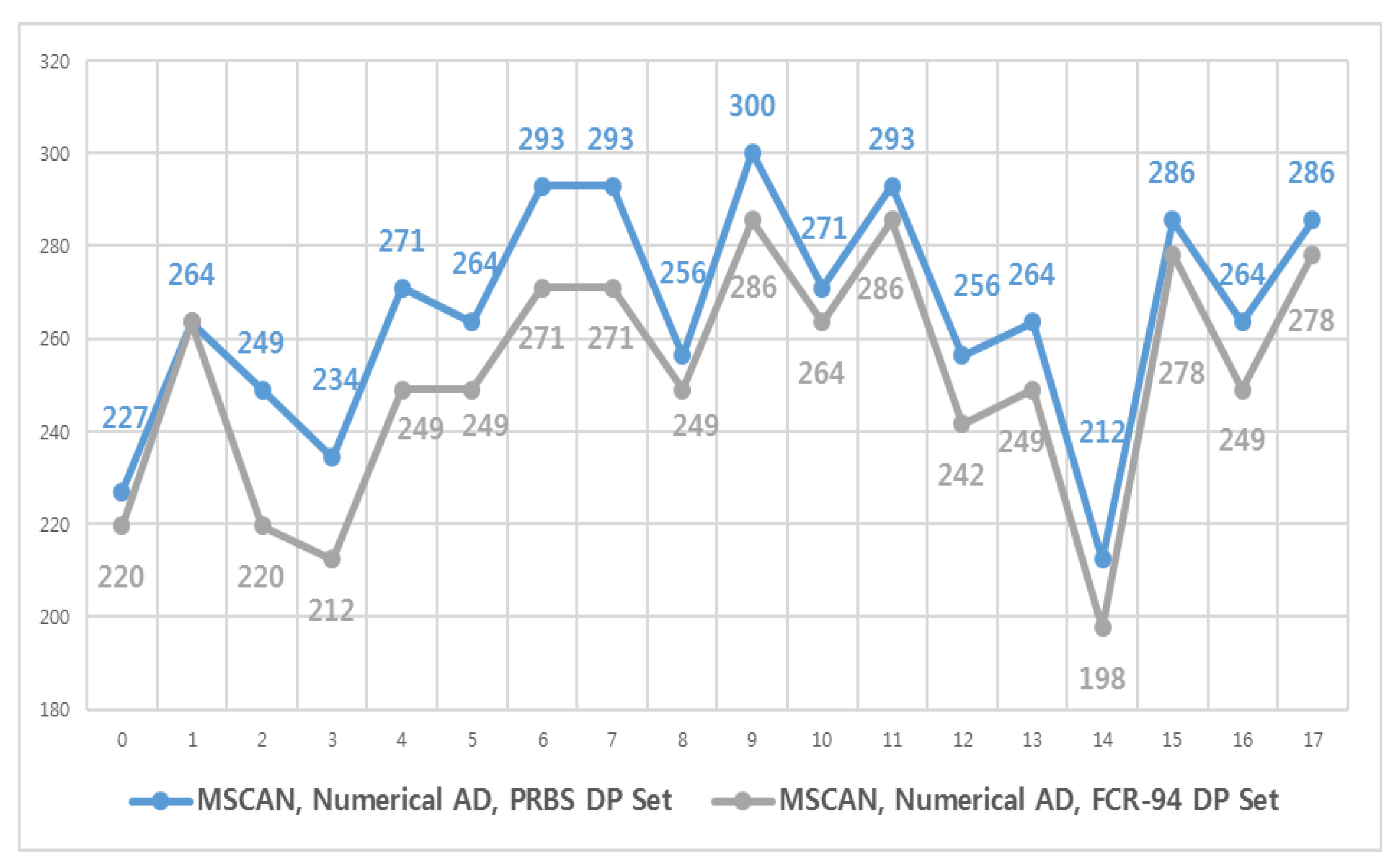### 2. Integration of Random and Fault Based DPs

A total of 62 DPs were deterministically generated after targeting interconnect fault models as discussed in Section 3. There are a total of 94 DPs for both fault-based DPs and critical random DPs. We will call these DPs as Fault-Critical-Random-94 (FCR-94) for convenience.

### 3. Timing Margins with a FCR-94 DP set

Each DP in a FCR-94 DP set was used as a data background pattern in the margin tests. MSCAN algorithm was repetitively executed for each DP of the FCR-94 DP set. In order to demonstrate the effectiveness of FCR-94 DPs, the test time was adjusted to be less than the execution test time of PRBS DPs in Section 4 by reducing the number of address bits by 8 (total 20 bits, row: 13 bits, column: 7 bits).

Fig. 6 shows the read margins of the PRBS DP set and the FCR-94 DP set. The write margin graph was not shown here since not many variations were observed between two DPs. For the read margin, the maximum reduction was 29.3 ps observed at DG 2. The margins of all DGs except DG 1 were decreased. For the write margin, 3 DG margins (DG 15, 16, 17) were reduced, and the maximum reduction was made at DG 15 and 16 (43.9 ps).

## VI. Conclusion

In this paper, we described an investigation into the most influential causes of timing margin degradation in DRAMs. We confirmed that DPs were the major contributors to reducing timing margins. Testing with 2$^{20}$ addresses for each of the FCR-94 DP set impacted the timing margins more than those of large numbers of random DPs. In other words, it is more effective to test the most critical DPs with a large address range, rather than testing with countless random DPs, such as the PRBS DP set, to measure the worst-case timing margins.

A DP selection method is a subject for future study, perhaps done by investigating which noise sources are included from random DPs. To achieve this goal, theoretical and experimental works are needed to determine which noise effects are contained from the random DPs. It can lead to guidelines for the DP selection method.

### ACKNOWLEDGMENTS

This research was supported by MOTIE (Ministry of Trade, Industry & Energy) (10052875) and KSRC (Korea Semiconductor Research Consortium) support program for the development of the future semiconductor device, and Basic Science Research Program through the National Research Foundation of Korea (NRF) funded by the Ministry of Science, ICT & Future Planning (2017R1A2B2002325).

### REFERENCES

1
Keeth B., Baker R.J., Johnson B., Lin F., 2007, DRAM Circuit Design: Fundamental and High-Speed Topics 2nd ed., Wiley-IEEE Press2
Jacob B., Ng S.W, Wang D.T., 2007, Memory Systems: Cache, DRAM, Disk, 1st ed., Morgan Kaufmann3
Kim C., Lee H.-W., Song J., 2016, Memory Interfaces: Past, Present, and Future, IEEE Solid-State Circuits Magazine, Vol. 8, No. 2, pp. 23-344
Vollrath J., Schwizer J., Gnat M., Schneider R., Johnson B., 2006, DDR2 DRAM output timing optimization, 2006 IEEE International Workshop on Memory Technology, Design, and Testing (MTDT’06), Vol. design, No. and testing (mtdt’06), pp. 49-545
Lingambudi A., Vijay S., Becker W.D., Raghavendra P., Sethuraman S., Pullelli S., 2016, Improve timing margins on multi-rank DDR3 RDIMM using read-on die termination sequencing, 2016 IEEE Annual India Conference (INDICON), pp. 1-46
S , Sethuraman , Lingambudi A., Wright K., Saurabh A., Kim K.-H., Becker D., 2014, Vref optimization in DDR4 RDIMMs for improved timing margins, 2014 IEEE Electrical Design of Advanced Packaging & Systems Symposium (EDAPS), pp. 73-767
Gupta A., Kumar A., Chhabra M., 2011, Characterizing Pattern Dependent Delay Effects in DDR Memory Interfaces, 2011 Asian Test Symposium, pp. 425-4318
Kim D., Kim H., Eo Y., 2012, Analytical Eye-Diagram Determination for the Efficient and Accurate Signal Integrity Verification of Single Interconnect Lines, IEEE Trans. on Computer-Aided Design of Integrated Circuits and Systems, Vol. 31, No. 10, pp. 1536-15459
Querbach B., Puligundla S., Becerra D., Schoenborn Z.T., Chiang P., 2013, Comparison of hardware based and software based stress testing of memory IO interface, 2013 IEEE 56th International Midwest Symposium on Circuits and Systems (MWSCAS), pp. 637-64010
Kim Y., Kang S.C., Lee S.K., Jung U., Kim S.M., Lee B.H., 2016, Hot-Carrier Instability of nMOSFETs under Pseudorandom Bit Sequence Stress, IEEE Electron Device Letters, Vol. 37, No. 4, pp. 366-36811
Garcia-Mora D.M., Garcia-Huanaco J., Zuniga-Marquez V.J., Franco-Tinoco C.J., Yahyaei-Moayyed F., Unger K.S., 2018, Power Delivery Network Impedance Characterization for High Speed I/O Interfaces using PRBS Transmissions, IEEE Electromagnetic Compatibility Magazine, Vol. 7, No. 1, pp. 87-9112
van de Goor A.J., 1998, Testing Semiconductor Memories: Theory and Practice, 1st ed., John Wiley & Sons Inc.13
Ciletti M.D., 2010, Advanced Digital Design with the Verilog HDL, 2nd ed., Pearson14
van de Goor A.J., Schanstra I., 2002, Address and data scrambling: causes and impact on memory tests, Proc. First IEEE International Workshop on Electronic Design, Test and Applications, pp. 128-136## Author

Kiseok Lee received the B.S. degree in electrical and communication engineering from Hanyang Univer-sity, Korea, in 2013.

Now he is in Hanyang University, Korea, working toward the Ph.D. degree in electronic and communication engineering.

His works have focused on memory fault diagnostics and memory test pattern optimization.

His current interests are noise-inducing data patterns on very large scale integration (VLSI) circuits and systems.

Tan Li received the B.S. degree in communication engineering from Harbin Institute of Technology, China, in 2010.

Now he is in Hanyang University, Korea, working toward the Ph.D. degree in electronic and communication engineering.

His work has focused on DRAM power integrity analysis and very large scale integrated circuit (VLSI) design for test (DFT) implementation and methodologies.

Sanghyeon Baeg received the B.S. degree in electronic engineering from Hanyang University, Seoul, Korea, in 1986 and the M.S. and Ph.D. degrees in electrical and computer engineering from the University of Texas at Austin, Austin, in 1988 and 1991, respectively.

From 1994 to 1997, he was a Staff Researcher with Samsung Electronics Company, Kihung, Korea.

In 1995, he was dispatched to Samsung Semiconductor, Inc., San Jose, CA, and worked as a member of the Technical Staff.

In 1997, he joined Cisco Systems, Inc., San Jose, CA, and worked as a Hardware Engineer, Technical Leader, and Hardware Manager.

Since 2004, he has been working as a Professor with Hanyang University, Ansan, Korea, in the School of Electrical Engineering and Computer Science.

His work has focused on reliable computing, soft error, low-power contents addressable memory (CAM), and VLSI DFT implementation and methodologies.

He is the holder of many U.S. patents in these fields.

Dr. Baeg was the recipient of an Inventor Recognition Award from Semiconductor Research Cooperation in 1993.

He was an IEEE 1149.6 working group member in 2003.

He serves as the organizing member of the Institute of Semiconductor Test of Korea from 2012.